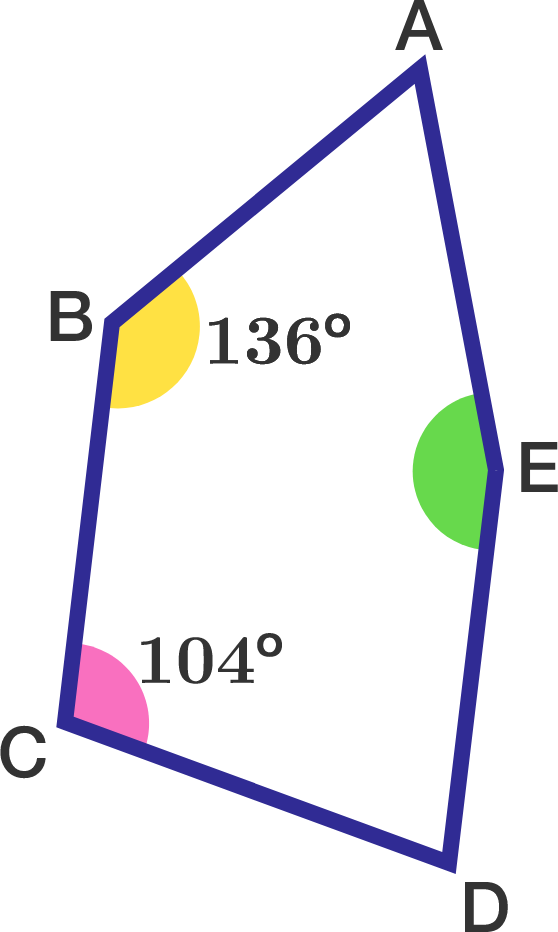# Angles in an equilateral pentagon

Geometry Level 4

Let $ABCDE$ be an equilateral convex pentagon such that $\angle ABC=136^\circ$ and $\angle BCD=104^\circ$. What is the measure (in degrees) of $\angle AED$?Note: An equilateral polygon is a polygon whose sides are all of the same length. It does not imply that all the internal angles are equal, nor that the polygon is cyclic.

×

Problem Loading...

Note Loading...

Set Loading...